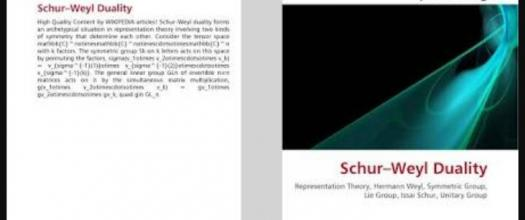# What Do You Know About Schur–weyl Duality?

10 Questions | Total Attempts: 104SettingsIn the representation theory branch of mathematics, Schur–Weyl duality relates unchangeable limited dimensional representations of the general symmetric and linear groups and gatherings. The mathematical theorem shapes a prototype circumstance in representation hypothesis including two sorts of symmetry that decide each other. To know more about Schur–Weyl duality, take this short quiz.

• 1.
Which theorem is used to prove the Schur–Weyl duality?
• A.

Double centralizer theorem

• B.

Fenz theorem

• C.

Optimum centralized theorem

• D.

Colligated theorem

• 2.
How many symmetries are involved in the puzzle?
• A.

2

• B.

4

• C.

6

• D.

8

• 3.
Which of these does S(k) represent?
• A.

Symmetry group

• B.

Symbol representation

• C.

Synchronization group

• D.

Keptic group

• 4.
Which of these does GL(n) represent?
• A.

Sequential number

• B.

Geometrical linear number

• C.

Geographical linear number

• D.

General linear number

• 5.
Which kind of information is the puzzle used for?
• A.

Scientific information

• B.

Mechanics information

• C.

Quantum information

• D.

Earth-related study

• E.

Energetics information

• 6.
What are the representations of the symmetric group?
• A.

Trivial representation and geometric representation

• B.

Sign representation and trivial representation

• C.

Mesh representation and sign representation

• D.

Fibonacci representation and sign representation

• 7.
How do you represent k=2 and n>1?
• A.

The space of two tensors decomposes into one part

• B.

The space of two tensor decomposes into two parts

• C.

The space of one tensor decomposes into halves

• D.

The space of two tensor remain unchanged

• 8.
What is the result of the trivial representation of S2?
• A.

Symmetric tensors

• B.

Asymmetric tensors

• C.

Both symmetric and asymmetric tensors

• D.

Tangential tensors

• 9.
Who discovered the puzzle?
• A.

Hermann Schur and Issai Weyl

• B.

Hermann Weyl and Issai Schur

• C.

Mattias Weyl and Isaac Schur

• D.

Matthew Weyl and John Schur

• 10.
What are the major groups used for determining the puzzle?
• A.

Linear and Symmetric

• B.

Geometric and Arithmetic

• C.

Finite and infinite

• D.

Inequality and Assymetric

Related TopicsBack to top